• 简而言之，树木
• 简而言之图
• 编码挑战

# Trees in a nutshell

• Root 是 最顶层节点。
• Edge 是链接 或两个节点之间的连接。
• Parent 是一个 节点，它与子节点有一条边。
• Child 是一个具有父节点的 节点。
• Leaf 是一个没有子节点的 节点。
• Height 是到叶子的最长路径的 长度。
• Depth 是到其根路径的长度。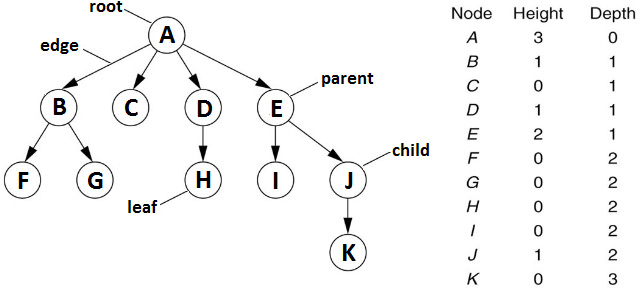## General tree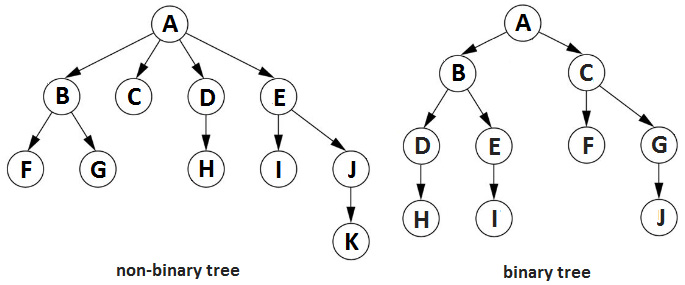``````private class Node {
private Node left;
private Node right;
private final T element;
public Node(T element) {
this.element = element;
this.left = null;
this.right = null;
}
public Node(Node left, Node right, T element) {
this.element = element;
this.left = left;
this.right = right;
}
// operations
}``````

### Knowing binary tree traversal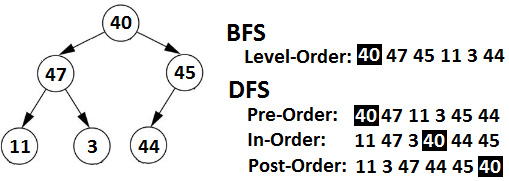#### Breadth-first Search (BFS) for trees

1. 从队列中弹出第一个节点作为当前节点。
2. 访问当前节点。
3. 如果当前节点有左节点，则将该左节点入队。
4. 如果当前节点有一个正确的节点，则将该正确的节点排入队列。
5. step 1 开始重复，直到队列为空。

``````private void printLevelOrder(Node node) {
Queue<Node> queue = new ArrayDeque<>();
while (!queue.isEmpty()) {
// Step 1
Node current = queue.poll();
// Step 2
System.out.print(" " + current.element);
// Step 3
if (current.left != null) {
}
// Step 4
if (current.right != null) {
}
}
}``````

#### Depth-first Search (DFS) for trees

Pre-Order遍历访问当前节点在其子节点之前，如下(root | left sub-tree | right sub-tree)

``````private void printPreOrder(Node node) {
if (node != null) {
System.out.print(" " + node.element);
printPreOrder(node.left);
printPreOrder(node.right);
}
}``````

``````private void printInOrder(Node node) {
if (node != null) {
printInOrder(node.left);
System.out.print(" " + node.element);
printInOrder(node.right);
}
}``````

Post-Order在其子节点之后访问当前节点，如下(left sub-树 | 右子树 | 根）：

``````private void printPostOrder(Node node) {
if (node != null) {
printPostOrder(node.left);
printPostOrder(node.right);
System.out.print(" " + node.element);
}
}``````

## Binary Search Tree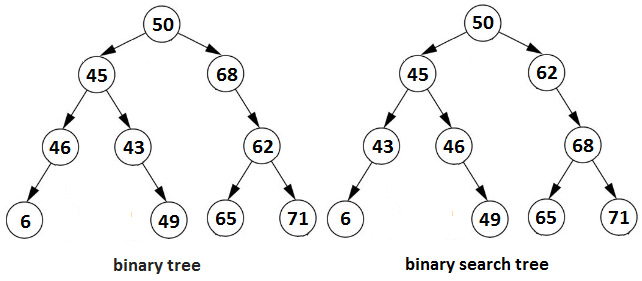## Balanced and unbalanced binary trees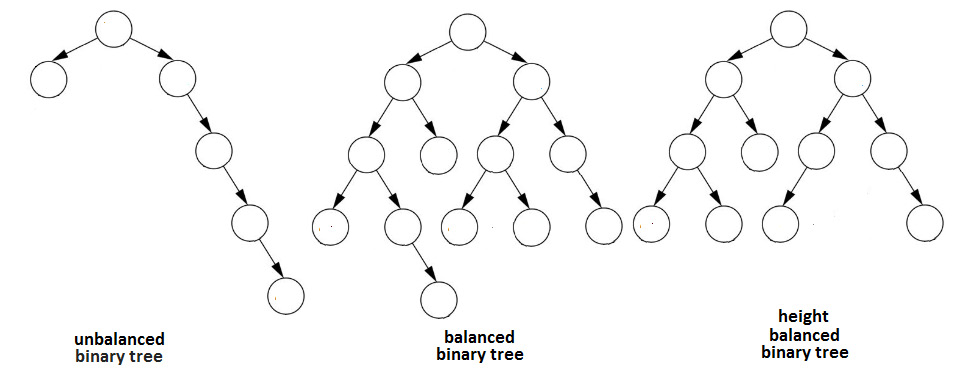### Red-Black tree

• 每个节点不是红色就是黑色
• 根节点始终为黑色
• 每片叶子（NULL）都是黑色的
• 红色节点的两个孩子都是黑色的
• 从节点到 NULL 节点的每条路径都有相同数量的黑色节点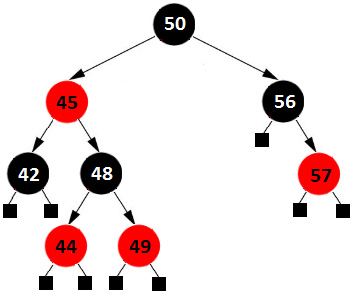### AVL tree

• 子树的高度最多可以相差 1。
• 节点（n）的平衡因子（BN）为-1、0或1，定义为高度(h) 区别：BN=h(right_subtree(n)) - h(left_subtree(n )) BN=h(left_subtree(< em class="italic">n)) - h(right_subtree(n))。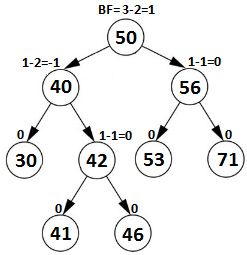AVL 树允许所有操作（插入、删除、查找最小值、查找最大值等）在 O(log n) 内执行，其中 n 是节点数。

## Complete binary tree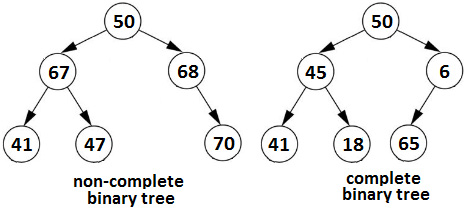## Full binary tree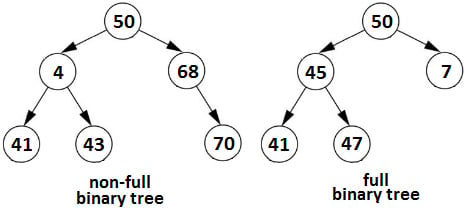## Perfect binary tree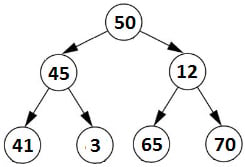## Binary Heaps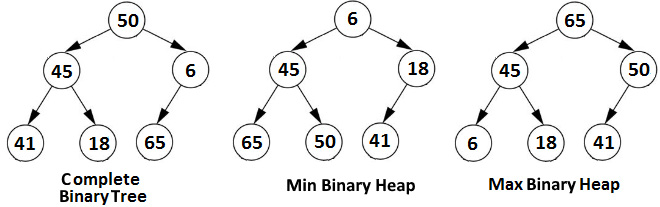• heap[(i - 1) / 2]：返回父节点
• heap[(2 * i) + 1]：返回左子节点
• heap[(2 * i) + 2]：返回右子节点

``````public class MaxHeap<T extends Comparable<T>> {
private static final int DEFAULT_CAPACITY = 5;
private int capacity;
private int size;
private T[] heap;
public MaxHeap() {
capacity = DEFAULT_CAPACITY;
this.heap = (T[]) Array.newInstance(
Comparable[].class.getComponentType(),DEFAULT_CAPACITY);
}
// operations
}``````

1. 从堆的末尾开始作为当前节点。
2. 当当前节点有父节点且父节点小于当前节点时，交换这些节点。

1. 从作为当前节点的堆的根开始。
2. 确定当前节点的子节点之间的最大节点。
3. 如果当前节点小于其最大的子节点，则交换这两个节点并从 step 2 开始重复；否则，没有别的事可做，所以停下来。

# Graphs in a nutshell

• Vertex 表示信息（例如，成员、狗或值）
• Edge 是两个顶点之间的连接或 关系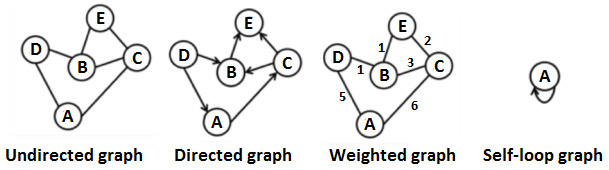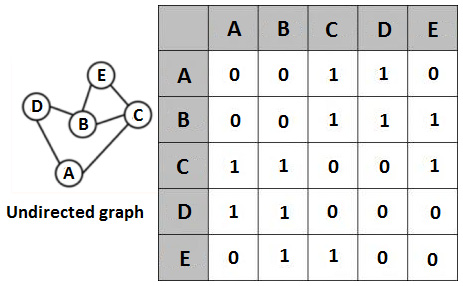``````public class Graph<T> {
// the vertices list
private final List<T> elements;
public Graph() {
this.elements = new ArrayList<>();
}
// operations
}``````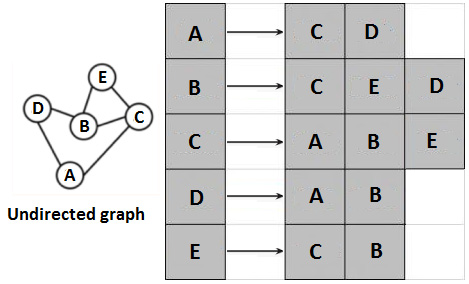``````public class Graph<T> {
// the adjacency list is represented as a map
public Graph() {
}
// operations
}``````

## Graph traversal

1. 将起始节点（当前节点）标记为已访问（将其添加到已访问节点的集合中）并将其添加到 BFS 队列中。
2. 从队列中弹出当前节点。
3. 访问当前节点。
4. 获取当前节点的相邻节点。
5. Loop the adjacent nodes. For each non-null and unvisited node, do the following:

一个。将其标记为已访问（将其添加到已访问节点的集合中）。

湾。将其添加到队列中。

6. 第 2 步 重复，直到队列为空。

### Depth-first Search (DFS) for graphs

#### DFS for graphs via recursion

1. 从当前节点（给定节点）开始，将当前节点标记为已访问（将其添加到已访问节点的集合中）。
2. 访问当前节点。
3. 通过递归遍历未访问的相邻顶点。

#### DFS for graphs – iterative implementation

DFS 算法的迭代实现依赖于`堆栈`。步骤如下：

1. 从当前节点（给定节点）开始，将当前节点推入`Stack`
2. While `Stack` is not empty, do the following:

一个。从 `Stack.` 中弹出当前节点

湾。访问当前节点。

C。将当前节点标记为已访问（将其添加到已访问节点的集合中）。

d。将未访问的相邻顶点推入 `Stack`

# Coding challenges

## Coding challenge 1 – Paths between two nodes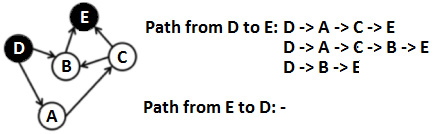``````public boolean isPath(T from, T to) {
Queue<T> queue = new ArrayDeque<>();
Set<T> visited = new HashSet<>();
// we start from the 'from' node
while (!queue.isEmpty()) {
T element = queue.poll();
for (T t : adjacents) {
if (t != null && !visited.contains(t)) {
// we reached the destination (the 'to' node)
if (t.equals(to)) {
return true;
}
}
}
}
}
return false;
}``````

## Coding challenge 2 – Sorted array to minimal BST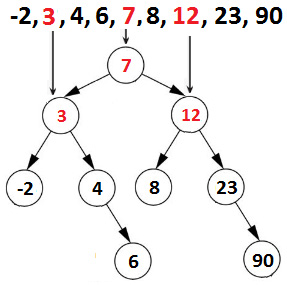1. 将数组的中间元素插入树中。
2. 将左子数组的元素插入左子树。
3. 将右子数组的元素插入右子树。
4. 触发递归调用。

``````public void minimalBst(T m[]) {
root = minimalBst(m, 0, m.length - 1);
}
private Node minimalBst(T m[], int start, int end) {
if (end < start) {
return null;
}
int middle = (start + end) / 2;
Node node = new Node(m[middle]);
nodeCount++;
node.left = minimalBst(m, start, middle - 1);
node.right = minimalBst(m, middle + 1, end);
return node;
}``````

## Coding challenge 3 – List per level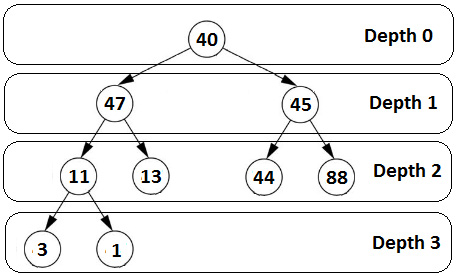``````public List<List<T>> fetchAllLevels() {
// each list holds a level
List<List<T>> allLevels = new ArrayList<>();
// first level (containing only the root)
Queue<Node> currentLevelOfNodes = new ArrayDeque<>();
List<T> currentLevelOfElements = new ArrayList<>();
while (!currentLevelOfNodes.isEmpty()) {
// store the current level as the previous level
Queue<Node> previousLevelOfNodes = currentLevelOfNodes;
// add level to the final list
// go to the next level as the current level
currentLevelOfNodes = new ArrayDeque<>();
currentLevelOfElements = new ArrayList<>();
// traverse all nodes on current level
for (Node parent : previousLevelOfNodes) {
if (parent.left != null) {
}
if (parent.right != null) {
}
}
}
return allLevels;
}``````

## Coding challenge 4 – sub-tree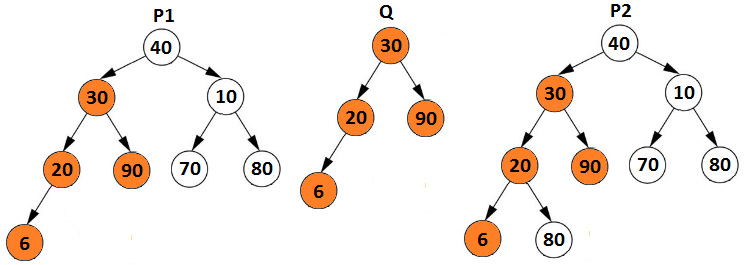• 如果 p 的根与 q 的根匹配 (p.root.element == q.root.element)，那么问题归结为检查q的右子树是否与p, 或者 q 的左子树是否与 的左子树相同p
• 如果 p 的根不匹配 q (p.root. element != q.root.element)，那么问题归结为检查 p 的左子树是否与 q, 或者 p 的右子树是否与 q 相同。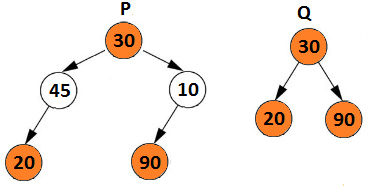``````public boolean isSubtree(BinaryTree q) {
return isSubtree(root, q.root);
}
private boolean isSubtree(Node p, Node q) {
if (p == null) {
return false;
}
// if the roots don't match
if (!match(p, q)) {
return (isSubtree(p.left, q) || isSubtree(p.right, q));
}
return true;
}
private boolean match(Node p, Node q) {
if (p == null && q == null) {
return true;
}
if (p == null || q == null) {
return false;
}
return (p.element == q.element
&& match(p.left, q.left)
&& match(p.right, q.right));
}``````

## Coding challenge 5 – Landing reservation system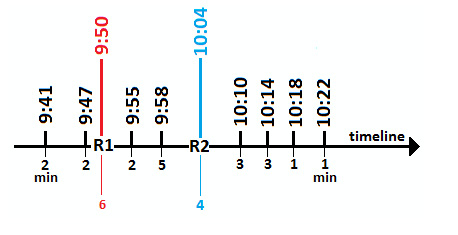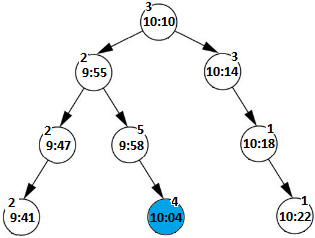``````long t1 = Duration.between(current.element.
plusMinutes(current.time), element).toMinutes();
long t2 = Duration.between(current.element,
element.plusMinutes(time)).toMinutes();
if (t1 <= 0 && t2 >= 0) {
// overlapping found
}``````

``````public class BinarySearchTree<Temporal> {
private Node root = null;
private class Node {
private Node left;
private Node right;
private final LocalTime element;
private final int time;
public Node(LocalTime element, int time) {
this.time = time;
this.element = element;
this.left = null;
this.right = null;
}
public Node(Node left, Node right,
LocalTime element, int time) {
this.time = time;
this.element = element;
this.left = left;
this.right = right;
}
}
public void insert(LocalTime element, int time) {
if (element == null) {
throw new IllegalArgumentException("...");
}
root = insert(root, element, time);
}
private Node insert(Node current,
LocalTime element, int time) {
if (current == null) {
return new Node(element, time);
}
long t1 = Duration.between(current.element.
plusMinutes(current.time), element).toMinutes();
long t2 = Duration.between(current.element,
element.plusMinutes(time)).toMinutes();
if (t1 <= 0 && t2 >= 0) {
System.out.println("Cannot reserve the runway at "
+ element + " for " + time + " minutes !");
return current;
}
if (element.compareTo(current.element) < 0) {
current.left = insert(current.left, element, time);
} else {
current.right = insert(current.right, element, time);
}
return current;
}
public void printInOrder() {
printInOrder(root);
}
private void printInOrder(Node node) {
if (node != null) {
printInOrder(node.left);
System.out.print(" " + node.element
+ "(" + node.time + ")");
printInOrder(node.right);
}
}
}``````

## Coding challenge 6 – Balanced binary tree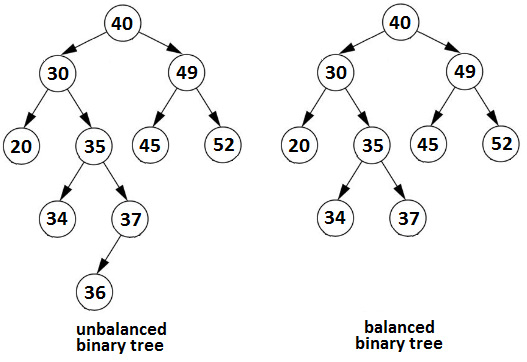``````public boolean isBalanced() {
return isBalanced(root);
}
private boolean isBalanced(Node root) {
if (root == null) {
return true;
}
if (Math.abs(height(root.left) - height(root.right)) > 1) {
return false;
} else {
return isBalanced(root.left) && isBalanced(root.right);
}
}
private int height(Node root) {
if (root == null) {
return 0;
}
return Math.max(height(root.left), height(root.right)) + 1;
}``````

``````public boolean isBalanced() {
return checkHeight(root) != Integer.MIN_VALUE;
}
private int checkHeight(Node root) {
if (root == null) {
return 0;
}
int leftHeight = checkHeight(root.left);
if (leftHeight == Integer.MIN_VALUE) {
return Integer.MIN_VALUE; // error
}
int rightHeight = checkHeight(root.right);
if (rightHeight == Integer.MIN_VALUE) {
return Integer.MIN_VALUE; // error
}
if (Math.abs(leftHeight - rightHeight) > 1) {
return Integer.MIN_VALUE; // pass error back
} else {
return Math.max(leftHeight, rightHeight) + 1;
}
}``````

## Coding challenge 7 – Binary tree is a BST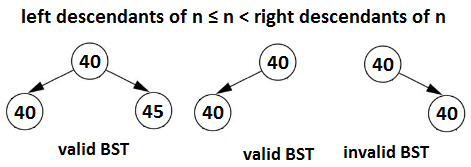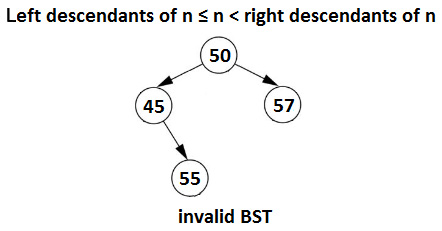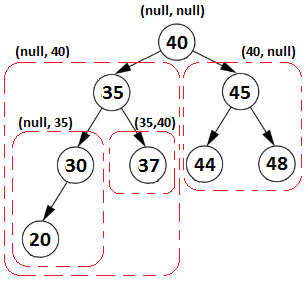``````public boolean isBinarySearchTree() {
return isBinarySearchTree(root, null, null);
}
private boolean isBinarySearchTree(Node node,
T minElement, T maxElement) {
if (node == null) {
return true;
}
if ((minElement != null &&
node.element.compareTo(minElement) <= 0)
|| (maxElement != null && node.element.
compareTo(maxElement) > 0)) {
return false;
}
if (!isBinarySearchTree(node.left, minElement, node.element)
|| !isBinarySearchTree(node.right,
node.element, maxElement)) {
return false;
}
return true;
}``````

## Coding challenge 8 – Successor node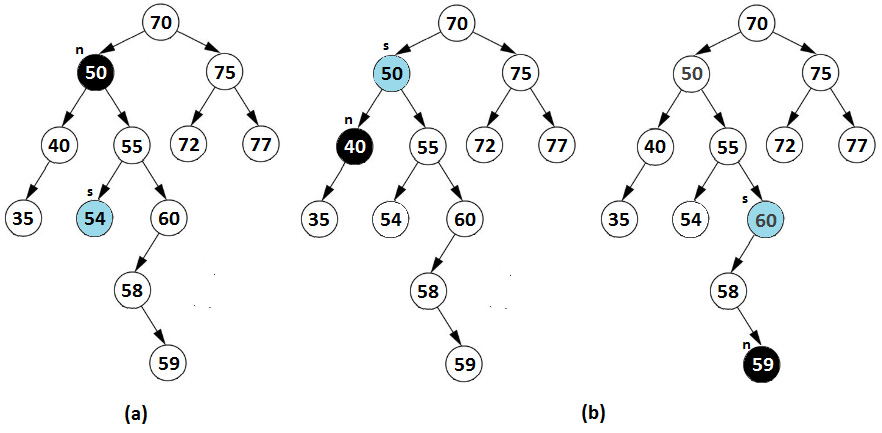(b) 的棘手子情况由上图中所示的右侧 BST 举例说明。当节点 n 不包含右子树且 n 是其父节点的右子节点时，则我们必须向上遍历，直到 n 成为其父级的左孩子。完成后，我们返回这个父级。例如，如果 n=59，则后继节点为 60。

``````Node inOrderSuccessor(Node n) {
if (n has a right sub-tree) {
return the leftmost child of right sub-tree
}
while (n is a right child of n.parent) {
n = n.parent; // traverse upwards
}
return n.parent; // parent has not been traversed
}``````

``````public void inOrderSuccessor() {
// choose the node
Node node = ...;
System.out.println("\n\nIn-Order:");
System.out.print("Start node: " + node.element);
node = inOrderSuccessor(node);
System.out.print(" Successor node: " + node.element);
}
private Node inOrderSuccessor(Node node) {
if (node == null) {
return null;
}
// case (a)
if (node.right != null) {
return findLeftmostNode(node.right);
}
// case (b)
while (node.parent != null && node.parent.right == node) {
node = node.parent;
}
return node.parent;
}``````

## Coding challenge 9 – Topological sort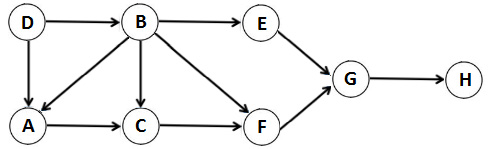``````public Stack<T> topologicalSort(T startElement) {
Set<T> visited = new HashSet<>();
Stack<T> stack = new Stack<>();
topologicalSort(startElement, visited, stack);
return stack;
}
private void topologicalSort(T currentElement,
Set<T> visited, Stack<T> stack) {
for (T t : adjacents) {
if (t != null && !visited.contains(t)) {
topologicalSort(t, visited, stack);
}
}
}
stack.push(currentElement);
}``````

## Coding challenge 10 – Common ancestor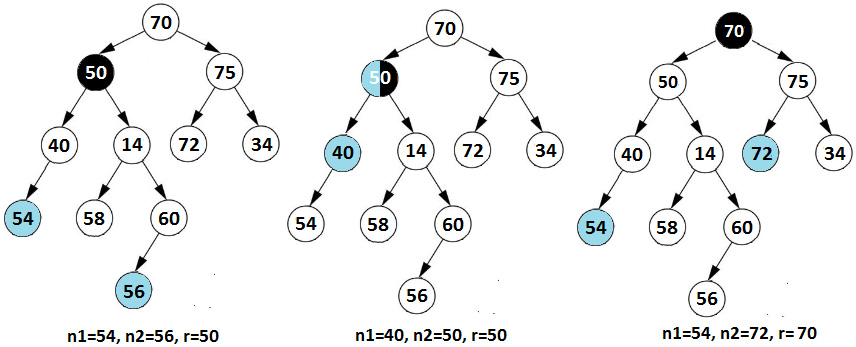• 如果根的子树包含 n1（并且不包含 n2<，则返回 n1 /em>)
• 如果根的子树包含 n2（并且不包含 n1<，则返回 n2 /em>)
• 如果 n1n2 都不在根的子树中，则返回 `null`
• 否则，它返回 n1n2 的共同祖先。

`commonAncestor(n.left, n1, n2)``commonAncestor(n.right, n1, n2)` 返回非空值，这意味着 n1n2 在不同的子树中并且 n 是共同的祖先。让我们从代码的角度来看：

``````public T commonAncestor(T e1, T e2) {
Node n1 = findNode(e1, root);
Node n2 = findNode(e2, root);
if (n1 == null || n2 == null) {
throw new IllegalArgumentException("Both nodes
must be present in the tree");
}
return commonAncestor(root, n1, n2).element;
}
private Node commonAncestor(Node root, Node n1, Node n2) {
if (root == null) {
return null;
}
if (root == n1 && root == n2) {
return root;
}
Node left = commonAncestor(root.left, n1, n2);
if (left != null && left != n1 && left != n2) {
return left;
}
Node right = commonAncestor(root.right, n1, n2);
if (right != null && right != n1 && right != n2) {
return right;
}
// n1 and n2 are not in the same sub-tree
if (left != null && right != null) {
return root;
} else if (root == n1 || root == n2) {
return root;
} else {
return left == null ? right : left;
}
}``````

## Coding challenge 11 – Chess knight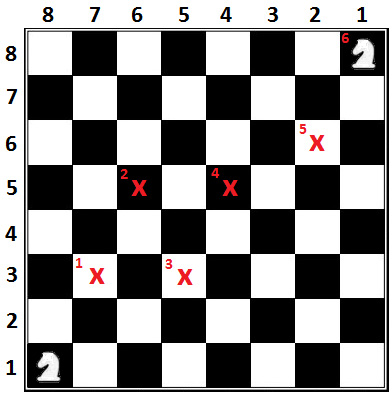1. 创建一个空队列。
2. 将起始单元排入队列，使其与自身的距离为 0。
3. As long as the queue is not empty, do the following:

一个。从队列中弹出下一个未访问的单元格。

湾。如果弹出的单元格是目标单元格，则返回其距离。

C。如果弹出的单元格不是目标单元格，则将此单元格标记为已访问，并通过将距离增加 1 将八个可能的移动中的每一个排入队列。

``````private int countknightMoves(Node startCell,
Node targetCell, int n) {
// store the visited cells
Set<Node> visited = new HashSet<>();
// create a queue and enqueue the start cell
Queue<Node> queue = new ArrayDeque<>();
while (!queue.isEmpty()) {
Node cell = queue.poll();
int r = cell.r;
int c = cell.c;
int distance = cell.distance;
// if destination is reached, return the distance
if (r == targetCell.r && c == targetCell.c) {
return distance;
}
// the cell was not visited
if (!visited.contains(cell)) {
// mark current cell as visited
// enqueue each valid movement into the queue
for (int i = 0; i < 8; ++i) {
// get the new valid position of knight from current
// position on chessboard and enqueue it in the queue
// with +1 distance
int rt = r + ROW[i];
int ct = c + COL[i];
if (valid(rt, ct, n)) {
queue.add(new Node(rt, ct, distance + 1));
}
}
}
}
// if path is not possible
return Integer.MAX_VALUE;
}
// Check if (r, c) is valid
private static boolean valid(int r, int c, int n) {
if (r < 0 || c < 0 || r >= n || c >= n) {
return false;
}
return true;
}``````

## Coding challenge 12 – Printing binary tree corners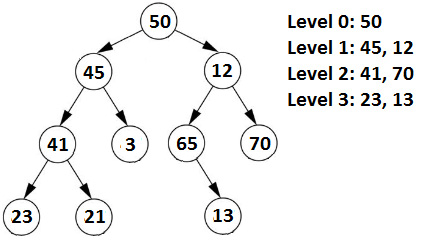``````public void printCorners() {
if (root == null) {
return;
}
Queue<Node> queue = new ArrayDeque<>();
int level = 0;
while (!queue.isEmpty()) {
// get the size of the current level
int size = queue.size();
int position = size;
System.out.print("Level: " + level + ": ");
level++;
// process all nodes present in current level
while (position > 0) {
Node node = queue.poll();
position--;
// if corner node found, print it
if (position == (size - 1) || position == 0) {
System.out.print(node.element + " ");
}
// enqueue left and right child of current node
if (node.left != null) {
}
if (node.right != null) {
}
}
// level done
System.out.println();
}
}``````

## Coding challenge 13 – Max path sum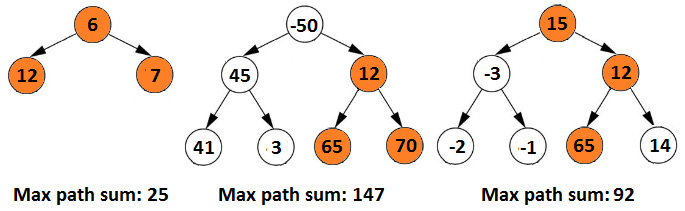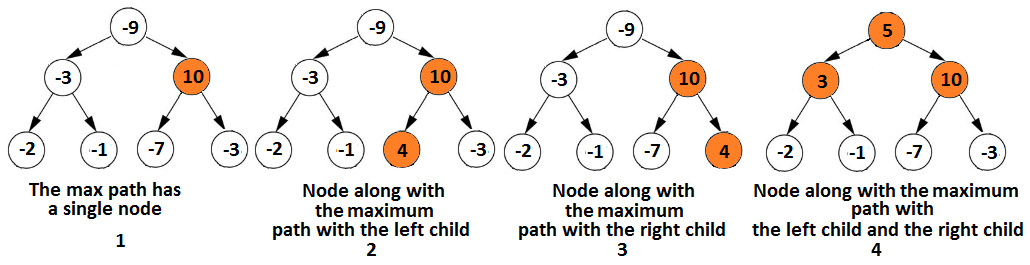1. 该节点是最大路径中的唯一节点
2. 该节点是其左子节点旁边的最大路径的一部分
3. 该节点是其右孩子旁边的最大路径的一部分
4. 该节点是其左右子节点旁边的最大路径的一部分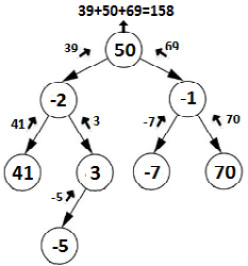• 41没有孩子，所以41加到max(0, 0), 41+max(0, 0)=41。
• 3只有左孩子，-5，所以3加到max(-5, 0), 3+max(-5, 0)=3。
• -2 被添加到 max(41, 3) 子树中，所以 -2+max(41, 3)=39。
• -7 没有子级，所以 max(0, 0) 加上 -7，-7+max(0, 0)=-7。
• 70没有孩子，所以max(0, 0)加上70，70+max(0, 0)=70。
• -1 被添加到 max(-7, 70) 个子树中，所以 -1+70=69。
• 50 被添加到左（39）和右（69）子树的最大值，所以 39+69+50=158（这是最大路径和）。

``````public int maxPathSum() {
maxPathSum(root);
return max;
}
private int maxPathSum(Node root) {
if (root == null) {
return 0;
}
// maximum of the left child and 0
int left = Math.max(0, maxPathSum(root.left));
// maximum of the right child and 0
int right = Math.max(0, maxPathSum(root.right));
// maximum at the current node (all four cases 1,2,3 and 4)
max = Math.max(max, left + right + root.element);
//return the maximum from left, right along with current
return Math.max(left, right) + root.element;
}``````

## Coding challenge 14 – Diagonal traversal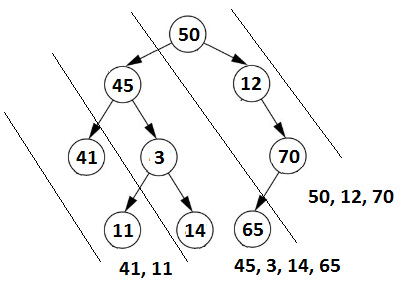### Recursion-based solution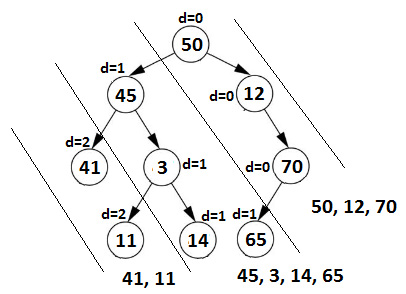``````// print the diagonal elements of given binary tree
public void printDiagonalRecursive() {
// map of diagonals
Map<Integer, List<T>> map = new HashMap<>();
// Pre-Order traversal of the tree and fill up the map
printDiagonal(root, 0, map);
// print the current diagonal
for (int i = 0; i < map.size(); i++) {
System.out.println(map.get(i));
}
}
// recursive Pre-Order traversal of the tree
// and put the diagonal elements in the map
private void printDiagonal(Node node,
int diagonal, Map<Integer, List<T>> map) {
if (node == null) {
return;
}
// insert the current node in the diagonal
if (!map.containsKey(diagonal)) {
map.put(diagonal, new ArrayList<>());
}
// increase the diagonal by 1 and go to the left sub-tree
printDiagonal(node.left, diagonal + 1, map);
// maintain the current diagonal and go
// to the right sub-tree
printDiagonal(node.right, diagonal, map);
}``````

### Iterative-based solution

``````(first diagonal)
Enqueue the root and all its right children
While the queue is not empty
Dequeue (let's denote it as A)
Print A
(next diagonal)
If A has a left child then enqueue it
(let's denote it as B)
Continue to enqueue all the right children of B``````

``````public void printDiagonalIterative() {
Queue<Node> queue = new ArrayDeque<>();
// mark the end of a diagonal via dummy null value
Node dummy = new Node(null);
// enqueue all the nodes of the first diagonal
while (root != null) {
root = root.right;
}
// enqueue the dummy node at the end of each diagonal
// loop while there are more nodes than the dummy
while (queue.size() != 1) {
Node front = queue.poll();
if (front != dummy) {
// print current node
System.out.print(front.element + " ");
// enqueue the nodes of the next diagonal
Node node = front.left;
while (node != null) {
node = node.right;
}
} else {
// at the end of the current diagonal enqueue the dummy
System.out.println();
}
}
}``````

## Coding challenge 15 – Handling duplicates in BSTs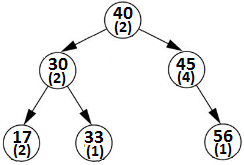``````private class Node {
private T element;
private int count;
private Node left;
private Node right;
private Node(Node left, Node right, T element) {
this.element = element;
this.left = left;
this.right = right;
this.count = 1;
}
}``````

``````private Node insert(Node current, T element) {
if (current == null) {
return new Node(null, null, element);
}
// START: Handle inserting duplicates
if (element.compareTo(current.element) == 0) {
current.count++;
return current;
}
// END: Handle inserting duplicates
...
}``````

``````private Node delete(Node node, T element) {
if (node == null) {
return null;
}
if (element.compareTo(node.element) < 0) {
node.left = delete(node.left, element);
} else if (element.compareTo(node.element) > 0) {
node.right = delete(node.right, element);
}
if (element.compareTo(node.element) == 0) {
// START: Handle deleting duplicates
if (node.count > 1) {
node.count--;
return node;
}
// END: Handle deleting duplicates
...
}``````

## Coding challenge 16 – Isomorphism of binary trees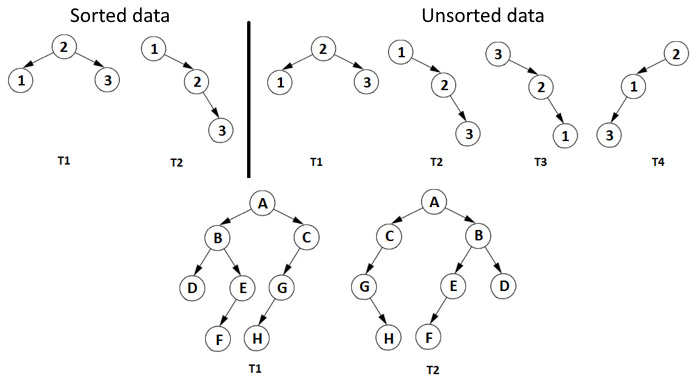1. 如果 T1T2`null`，则 它们是同构的，所以返回 `true。`
2. 如果 T1T2`null`，那么它们不是同构的，所以返回 `false.`
3. 如果 T1.data 不等于 T2.data, 那么它们不是同构的，所以返回 `假。`
4. 遍历T1的左子树和T2的左子树。
5. Traverse the right sub-tree of T1 and the right sub-tree of T2:

一个。如果T1T2的结构相同，则返回`true。`

湾。如果 T1T2 的结构不相同，那么我们检查一棵树（或子树）是否在镜像另一棵树树（子树），

6. 遍历T1的左子树和T2的右子树。
7. Traverse the right sub-tree of T1 and the left sub-tree of T2:

一个。如果结构被镜像，则返回 `true`;否则，返回 `false。`

``````private boolean isIsomorphic(Node treeOne, Node treeTwo) {
// step 1
if (treeOne == null && treeTwo == null) {
return true;
}
// step 2
if ((treeOne == null || treeTwo == null)) {
return false;
}
// step 3
if (!treeOne.element.equals(treeTwo.element)) {
return false;
}
// steps 4, 5, 6 and 7
return (isIsomorphic(treeOne.left, treeTwo.right)
&& isIsomorphic(treeOne.right, treeTwo.left)
|| isIsomorphic(treeOne.left, treeTwo.left)
&& isIsomorphic(treeOne.right, treeTwo.right));
}
.``````

## Coding challenge 17 – Binary tree right view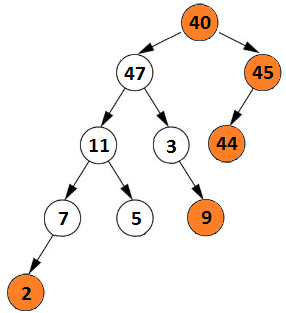• 40、47、45、11、3、44、7、 5、9、2

``````private void printRightViewIterative(Node root) {
if (root == null) {
return;
}
// enqueue root node
Queue<Node> queue = new ArrayDeque<>();
Node currentNode;
while (!queue.isEmpty()) {
// number of nodes in the current level is the queue size
int size = queue.size();
int i = 0;
// traverse each node of the current level and enqueue its
// non-empty left and right child
while (i < size) {
i++;
currentNode = queue.poll();
// if this is last node of current level just print it
if (i == size) {
System.out.print(currentNode.element + " ");
}
if (currentNode.left != null) {
}
if (currentNode.right != null) {
}
}
}
}``````

## Coding challenge 18 – kth largest element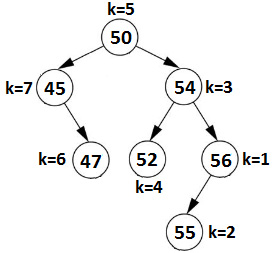``````public void kthLargest(int k) {
kthLargest(root, k);
}
private int c;
private void kthLargest(Node root, int k) {
if (root == null || c >= k) {
return;
}
kthLargest(root.right, k);
c++;
// we found the kth largest value
if (c == k) {
System.out.println(root.element);
}
kthLargest(root.left, k);
}``````

## Coding challenge 19 – Mirror binary tree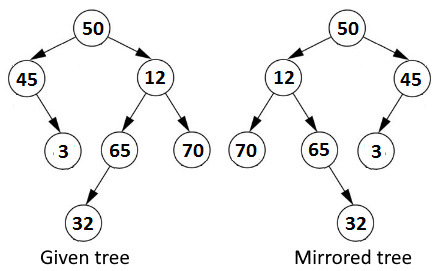### Mirroring the given tree in a new tree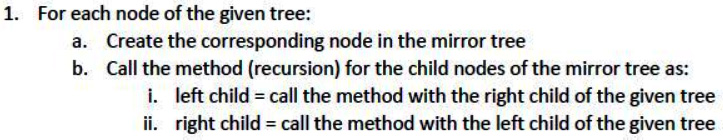``````private Node mirrorTreeInTree(Node root) {
if (root == null) {
return null;
}
Node node = new Node(root.element);
node.left = mirrorTreeInTree(root.right);
node.right = mirrorTreeInTree(root.left);
return node;
}``````

### Mirroring the given tree in place

1. 镜像给定树的左子树。
2. 镜像给定树的右子树。
3. 交换左右子树（交换它们的指针）。

``````private void mirrorTreeInPlace(Node node) {
if (node == null) {
return;
}
Node auxNode;
mirrorTreeInPlace(node.left);
mirrorTreeInPlace(node.right);
auxNode = node.left;
node.left = node.right;
node.right = auxNode;
}``````

## Coding challenge 20 – Spiral-level order traversal of a binary tree

• 奇数级别应该从左到右打印，偶数级别应该从右到左打印。
• 奇数级别应该从右到左打印，偶数级别应该从左到右打印。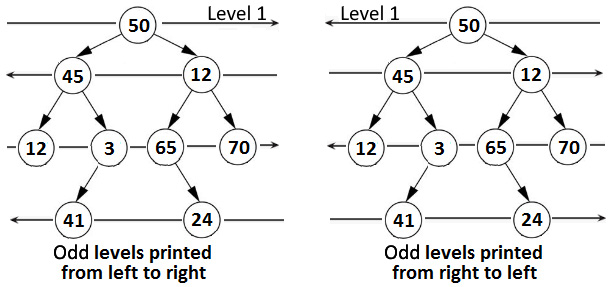### Recursive approach

``````public void spiralOrderTraversalRecursive() {
if (root == null) {
return;
}
int level = 1;
boolean flip = false;
// as long as printLevel() returns true there
// are more levels to print
while (printLevel(root, level++, flip = !flip)) {
// there is nothing to do
};
}
// print all nodes of a given level
private boolean printLevel(Node root,
int level, boolean flip) {
if (root == null) {
return false;
}
if (level == 1) {
System.out.print(root.element + " ");
return true;
}
if (flip) {
// process left child before right child
boolean left = printLevel(root.left, level - 1, flip);
boolean right = printLevel(root.right, level - 1, flip);
return left || right;
} else {
// process right child before left child
boolean right = printLevel(root.right, level - 1, flip);
boolean left = printLevel(root.left, level - 1, flip);
return right || left;
}
}``````

### Iterative approach

``````private void printSpiralTwoStacks(Node node) {
if (node == null) {
return;
}
// create two stacks to store alternate levels
Stack<Node> rl = new Stack<>(); // right to left
Stack<Node> lr = new Stack<>(); // left to right
// Push first level to first stack 'rl'
rl.push(node);
// print while any of the stacks has nodes
while (!rl.empty() || !lr.empty()) {
// print nodes of the current level from 'rl'
// and push nodes of next level to 'lr'
while (!rl.empty()) {
Node temp = rl.peek();
rl.pop();
System.out.print(temp.element + " ");
if (temp.right != null) {
lr.push(temp.right);
}
if (temp.left != null) {
lr.push(temp.left);
}
}
// print nodes of the current level from 'lr'
// and push nodes of next level to 'rl'
while (!lr.empty()) {
Node temp = lr.peek();
lr.pop();
System.out.print(temp.element + " ");
if (temp.left != null) {
rl.push(temp.left);
}
if (temp.right != null) {
rl.push(temp.right);
}
}
}
}``````

## Coding challenge 21 – Nodes at a distance k from leafs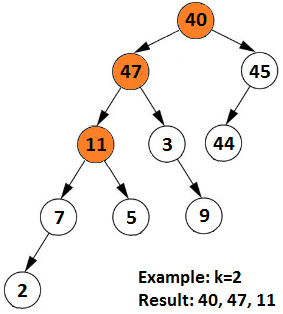• 节点 40 与叶 44 的距离为 2。
• 节点 47 与叶子 9 和叶子 5 的距离为 2。
• 节点 11 与叶子 2 的距离为 2。

• 距叶节点距离为 1 的节点为 3、11、7 和 45。
• 距叶节点距离为 2 的节点为 11、47 和 40。
• 离叶节点距离为 3 的节点是 40 和 47。
• 距离叶节点 4 的节点是 40 。

``````private void leafDistance(Node node,
List<Node> pathToLeaf, Set<Node> nodesAtDist, int dist) {
if (node == null) {
return;
}
// for each leaf node, store the node at distance 'dist'
if (isLeaf(node) && pathToLeaf.size() >= dist) {
return;
}
// add the current node into the current path
// go  to left and right subtree via recursion
leafDistance(node.left, pathToLeaf, nodesAtDist, dist);
leafDistance(node.right, pathToLeaf, nodesAtDist, dist);
// remove the current node from the current path
pathToLeaf.remove(node);
}
private boolean isLeaf(Node node) {
return (node.left == null && node.right == null);
}``````

## Coding challenge 22 – Pair for a given sum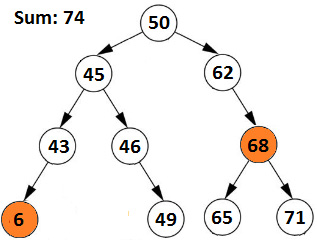``````public boolean findPairSum(int sum) {
return findPairSum(root, sum, new HashSet());
}
private static boolean findPairSum(Node node,
int sum, Set<Integer> set) {
// base case
if (node == null) {
return false;
}
// find the pair in the left subtree
if (findPairSum(node.left, sum, set)) {
return true;
}
// if pair is formed with current node then print the pair
if (set.contains(sum - node.element)) {
System.out.print("Pair (" + (sum - node.element) + ", "
+ node.element + ") = " + sum);
return true;
} else {
}
// find the pair in the right subtree
return findPairSum(node.right, sum, set);
}``````

• 前序遍历（最左边的节点是第一个访问的节点）
• 逆序遍历（最右边的节点是第一个访问的节点）

• if (最小值 + 最大值) sum，然后转到下一个 minimum（前向有序遍历返回的下一个节点）。
• if (最小 + 最大) > sum，然后进入下一个maximum（逆序遍历返回的下一个节点）。
• 如果 (minimum + maximum) = sum，则返回 `true。`

• if (最小值 + 最大值) sum，然后我们通过 Forward In-Order 遍历到弹出节点的右子树。这就是我们如何找到下一个最大元素的方法。
• if (最小 + 最大) > sum，然后我们通过 Reverse In-Order 遍历到弹出节点的左子树。这就是我们如何找到下一个最小元素的方法。
• 如果 (minimum + maximum) = sum，那么我们找到了一对验证给定 总和

``````public boolean findPairSumTwoStacks(int sum) {
return findPairSumTwoStacks(root, sum);
}
private static boolean findPairSumTwoStacks(
Node node, int sum) {
Stack<Node> fio = new Stack<>(); // fio - Forward In-Order
Stack<Node> rio = new Stack<>(); // rio - Reverse In-Order
Node minNode = node;
Node maxNode = node;
while (!fio.isEmpty() || !rio.isEmpty()
|| minNode != null || maxNode != null) {
if (minNode != null || maxNode != null) {
if (minNode != null) {
fio.push(minNode);
minNode = minNode.left;
}
if (maxNode != null) {
rio.push(maxNode);
maxNode = maxNode.right;
}
} else {
int elem1 = fio.peek().element;
int elem2 = rio.peek().element;
if (fio.peek() == rio.peek()) {
break;
}
if ((elem1 + elem2) == sum) {
System.out.print("\nPair (" + elem1 + ", "
+ elem2 + ") = " + sum);
return true;
}
if ((elem1 + elem2) < sum) {
minNode = fio.pop();
minNode = minNode.right;
} else {
maxNode = rio.pop();
maxNode = maxNode.left;
}
}
}
return false;
}``````

## Coding challenge 23 – Vertical sums in a binary tree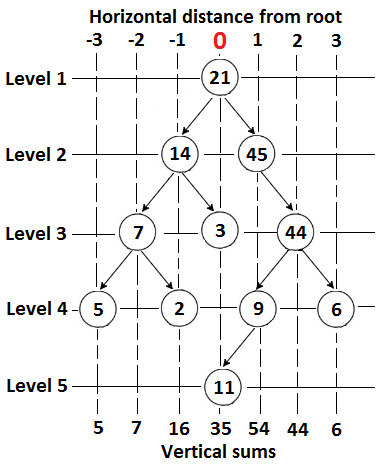``````private void verticalSum(Node root,
Map<Integer, Integer> map, int dist) {
if (root == null) {
return;
}
if (!map.containsKey(dist)) {
map.put(dist, 0);
}

map.put(dist, map.get(dist) + root.element);
// or in functional-style
/*
BiFunction <Integer, Integer, Integer> distFunction
= (distOld, distNew) -> distOld + distNew;
map.merge(dist, root.element, distFunction);
*/
// decrease horizontal distance by 1 and go to left
verticalSum(root.left, map, dist - 1);
// increase horizontal distance by 1 and go to right
verticalSum(root.right, map, dist + 1);
}``````

## Coding challenge 23 – Converting a max heap into a min heap

Solution：这个问题的解决方案受到Heap Sort算法的启发（这个算法在第 14 章，排序和搜索）。

1. 从给定数组的最右边、最底部的节点（最后一个内部节点）开始。
2. Heapify 所有节点通过自底向上技术。

``````public static void convertToMinHeap(int[] maxHeap) {
// build heap from last node to all
// the way up to the root node
int p = (maxHeap.length - 2) / 2;
while (p >= 0) {
heapifyMin(maxHeap, p--, maxHeap.length);
}
}
// heapify the node at index p and its two direct children
private static void heapifyMin(int[] maxHeap,
int p, int size) {
// get left and right child of node at index p
int left = leftChild(p);
int right = rightChild(p);
int smallest = p;
// compare maxHeap[p] with its left and
// right child and find the smallest value
if ((left < size) && (maxHeap[left] < maxHeap[p])) {
smallest = left;
}
if ((right < size)
&& (maxHeap[right] < maxHeap[smallest]))  {
smallest = right;
}
// swap 'smallest' with 'p' and heapify
if (smallest != p) {
swap(maxHeap, p, smallest);
heapifyMin(maxHeap, smallest, size);
}
}
/* Helper methods */
private static int leftChild(int parentIndex) {
return (2 * parentIndex + 1);
}
private static int rightChild(int parentIndex) {
return (2 * parentIndex + 2);
}
// utility function to swap two indices in the array
private static void swap(int heap[], int i, int j) {
int aux = heap[i];
heap[i] = heap[j];
heap[j] = aux;
}``````

## Coding challenge 24 – Finding out whether a binary tree is symmetric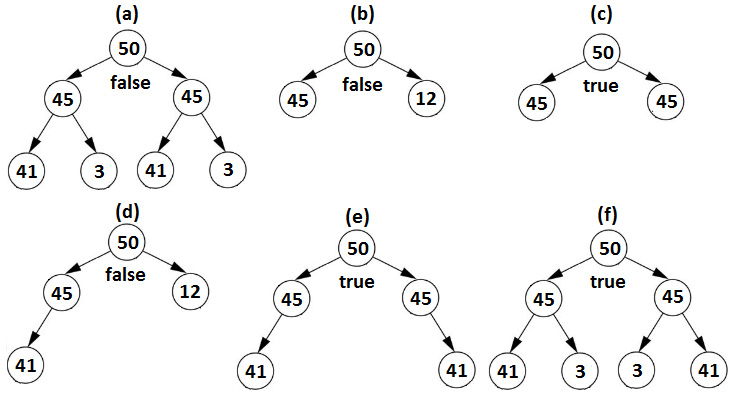1. 根节点的元素是相同的。
2. 左树的左子树和右树的右子树必须是镜像。
3. 左树的右子树和右树的左子树必须是镜像。

``````private boolean isSymmetricRecursive(
Node leftNode, Node rightNode) {
boolean result = false;
// empty trees are symmetric
if (leftNode == null && rightNode == null) {
result = true;
}
// conditions 1, 2, and 3 from above
if (leftNode != null && rightNode != null) {
result = (leftNode.element.equals(rightNode.element))
&& isSymmetricRecursive(leftNode.left, rightNode.right)
&& isSymmetricRecursive(leftNode.right, rightNode.left);
}
return result;
}``````

``````public boolean isSymmetricIterative() {
boolean result = false;
queue.offer(root.left);
queue.offer(root.right);
while (!queue.isEmpty()) {
Node left = queue.poll();
Node right = queue.poll();
if (left == null && right == null) {
result = true;
} else if (left == null || right == null
|| left.element != right.element) {
result = false;
break;
} else {
queue.offer(left.left);
queue.offer(right.right);
queue.offer(left.right);
queue.offer(right.left);
}
}
return result;
}``````

## Coding challenge 25 – Connecting n ropes at the minimum cost

1. 按绳索的长度降序排列。
2. 连接前两条绳索并更新部分最低成本。
3. 将前两条绳索替换为生成的绳索。
4. step 1 重复，直到剩下一根绳子（连接所有绳子的结果）。

1. 从绳索长度数组 (O(log n)) 创建最小二进制堆。
2. 轮询最小二叉堆的根，这将为我们提供最小的绳索 (O(log n))。
3. 再次轮询根，这将为我们提供第二小的绳索 (O(log n))。
4. 连接两条绳索（总结它们的长度）并将结果放回最小二进制堆。
5. step 2 重复，直到剩下一根绳子（连接所有绳子的结果）。

``````public int minimumCost(int[] ropeLength) {
if (ropeLength == null) {
return -1;
}
// add the lengths of the ropes to the heap
for (int i = 0; i < ropeLength.length; i++) {
}
int totalLength = 0;
while (size() > 1) {
int l1 = poll();
int l2 = poll();
totalLength += (l1 + l2);
}
}``````

• AVL 树（本书附带的代码中提供了简要说明和实现）
• 红黑树（本书附带的代码中提供了简要描述和实现）
• Dijkstra 算法
• Rabin-Karp 子串搜索
• 贝尔曼福特算法
• Floyd-Warshall 算法
• 区间树
• 最小生成树
• B树
• 二分图
• 图形着色
• P、NP 和 NP 完全
• 组合和概率
• 常用表达
• 一个*